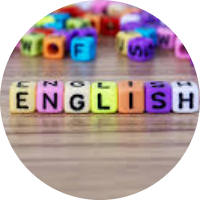# Mathematics Magic

This set of MCQs helps you brush up on important math topics and prepare you to dive into skill practice.

Start Quiz

The volume of a rectangular box is 472 cubic inches. The height of the box is 4 inches. The width of the box is 3 inches less than the length. Which equation can you use to find w, the width of the box in inches?

w(w+3) = 472

4w(w-3) = 472

4w(w+3) = 472

4w^2 = 472

Three friends share the cost of a meal. The check is \$55.60.They decide to leave a 20% tip for the waiter. One friend pays \$20,and the other two split the rest of the cost equally. Which equation can be used to find the amount, p, that each of the remaining friends pays?

2p+10 = 56.72

2p+20 = 66.72

2p+10 = 66.72

p+10 = 66.72

Each side of a sandbox is 5 feet long. It will cost \$1.59 per square foot to replace the sand in the sandbox. What would be the total cost to replace the sand?

39.75

39.25

49.75

29.75

A fence will be built to enclose a 100m X 70m field. It will need a post at each corner and one every 5m. How many posts are needed?

100

70

68

75

A bracelet is to be made from 2 red and 5 blue beads. How many different bracelets can be made?

3

1

2

4

A square with side lengths of 15 cm is reduced by a scale factor of 0.8. Determine the side lengths of the new square.

4

12.25

18.75

12

A plush toy is a scale model of a horse in which 1 cm represents 3 cm. The head-on the model is 15 cm long How long is the head of the real horse?

30

10

15

60

Larry runs a dog-walking service. He charges \$5/h plus a flat fee of \$6. One day, he earned \$16. Determine which equation represents this.

16h - 5 = 600

16h + 5 = 600

5h + 6 = 16

5h - 6 = 16

Quiz/Test Summary
Title: Mathematics Magic
Questions: 8
Contributed by: